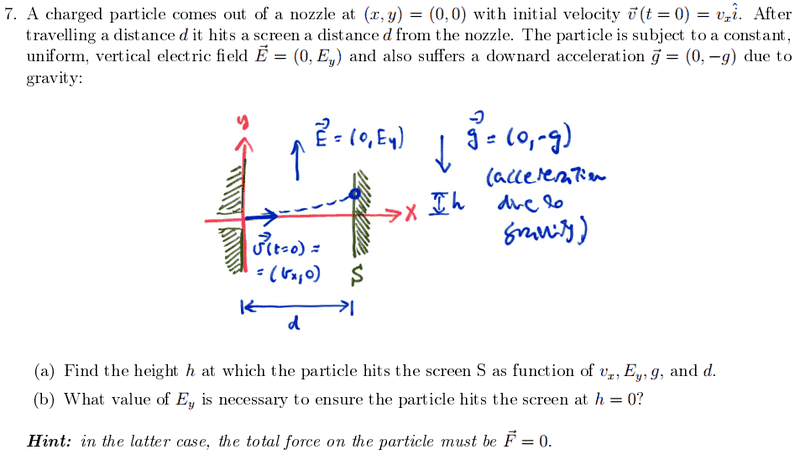# Motion of a particle in eletric field

• Taylor_1989

## Homework Statement

Hi guys. Just wondering if anyone could help me with Q7 part a) on the pdf I have attached below. I think my working are not correct but I can't see other way. If I add 6. and 7. together I would get an ans in terms of what the question is asking but I am not sure if that is correct?[ note: cropped image inserted by moderator ]

## Homework Equations

1.$$y=y_0+v_{0y}(t)+\frac{1}{2}at^2$$
2.$$E_y=\frac{F_y}{q}$$
3. $$F_y=-mg$$

## The Attempt at a Solution

4.$$y_0=0, v_y=0 ,\uparrow=+, v_{x0}$$
5.$$E_y=\frac{-mg}{q}, g=\frac{E_yq}{m}$$

So by subbing in the 4 and 5 values into 1 I get two equations as shown below:
6.$$y=-\frac{1}{2}g(\frac{d}{v_{x0}})^2$$
and

7.
$$y=-\frac{1}{2}(\frac{E_yq}{m})(\frac{d}{v_{x0}})^2$$

[/B]

#### Attachments

• ph322_problems.pdf
621.4 KB · Views: 118

#3 only follows from #2 in a specific situation - does that situation apply for 7a?

You should start from a free body diagram.
Use: ##\sum \vec F = m\vec a## ... divide into components and use suvat equations. Y
ou've done something similar when you learned about ballistics.

#3 only follows from #2 in a specific situation - does that situation apply for 7a?

You should start from a free body diagram.
Use: ##\sum \vec F = m\vec a## ... divide into components and use suvat equations. Y
ou've done something similar when you learned about ballistics.

I am slight lost to what you mean. I have used suvat, in the x and y. Could you please expand on this. From the picture the only accleration acting is in the horizontal no acceleration in the vertical. So the horizontal equation looks like so:

$$d=v_xt$$

Last edited:
the only accleration acting is in the horizontal no acceleration in the vertical
You meant the other way around, yes?
What forces act in the vertical? What is the net vertical force? What vertical acceleration results?

I am slight lost to what you mean. I have used suvat, in the x and y. Could you please expand on this.
Identify the vertical forces and write out Newton's second law accordingly.
From the picture the only acceleration acting is in the [vertical] no acceleration in the [horizontal]. So the horizontal equation looks like so: $$d=v_xt$$
So, in the horizontal direction, Newton's law says: ##\sum F_x = 0 = ma_x: v_x(0)=u, x(0)=0##
... when you solve that, you get ##a_x=0, v_x(t)=u, \implies x(t)=ut##

Good ... so what are the forces in the vertical direction?
##\sum F_y = ? = ma_y : v_y(0)=?, y(0)=?## ... fill in the question marks.

Identify the vertical forces and write out Newton's second law accordingly.
So, in the horizontal direction, Newton's law says: ##\sum F_x = 0 = ma_x: v_x(0)=u, x(0)=0##
... when you solve that, you get ##a_x=0, v_x(t)=u, \implies x(t)=ut##

Good ... so what are the forces in the vertical direction?
##\sum F_y = ? = ma_y : v_y(0)=?, y(0)=?## ... fill in the question marks.
Ok so the net force that act of the particle is:
$$F_t=E_yq-g=ma_y$$

using the equation for height:

$$y=v_{y0}t+\frac{1}{2}a_yt^2$$

now at $$t=0, v_{y0}=0$$

therfore my equation becomes:

$$y=\frac{1}{2}at^2$$

subbing in my equations for $$a_y ,t$$

I get:

$$y=(\frac{1}{2})(\frac{E_yq-g}{m})(\frac{d^2}{v_x^2})$$

Ok so the net force that act of the particle is:
$$F_t=E_yq-g=ma_y$$

using the equation for height:

$$y=v_{y0}t+\frac{1}{2}a_yt^2$$

now at $$t=0, v_{y0}=0$$

therfore my equation becomes:

$$y=\frac{1}{2}at^2$$

subbing in my equations for $$a_y ,t$$

I get:

$$y=(\frac{1}{2})(\frac{E_yq-g}{m})(\frac{d^2}{v_x^2})$$
Looks right.

Just like to say thank for the guidance.

Ok so the net force that act of the particle is:
$$F_t=E_yq-g=ma_y$$
Force of gravity is usually written ##mg##.

Note: it is usually best practise to use the variable names in the question.
##d = uT## and ##2h = aT^2## where T is time between plates... maybe?

Force of gravity is usually written ##mg##.

Note: it is usually best practise to use the variable names in the question.
##d = uT## and ##2h = aT^2## where T is time between plates... maybe?
yes sorry, I notice that when i looked back. But i could not edit it.